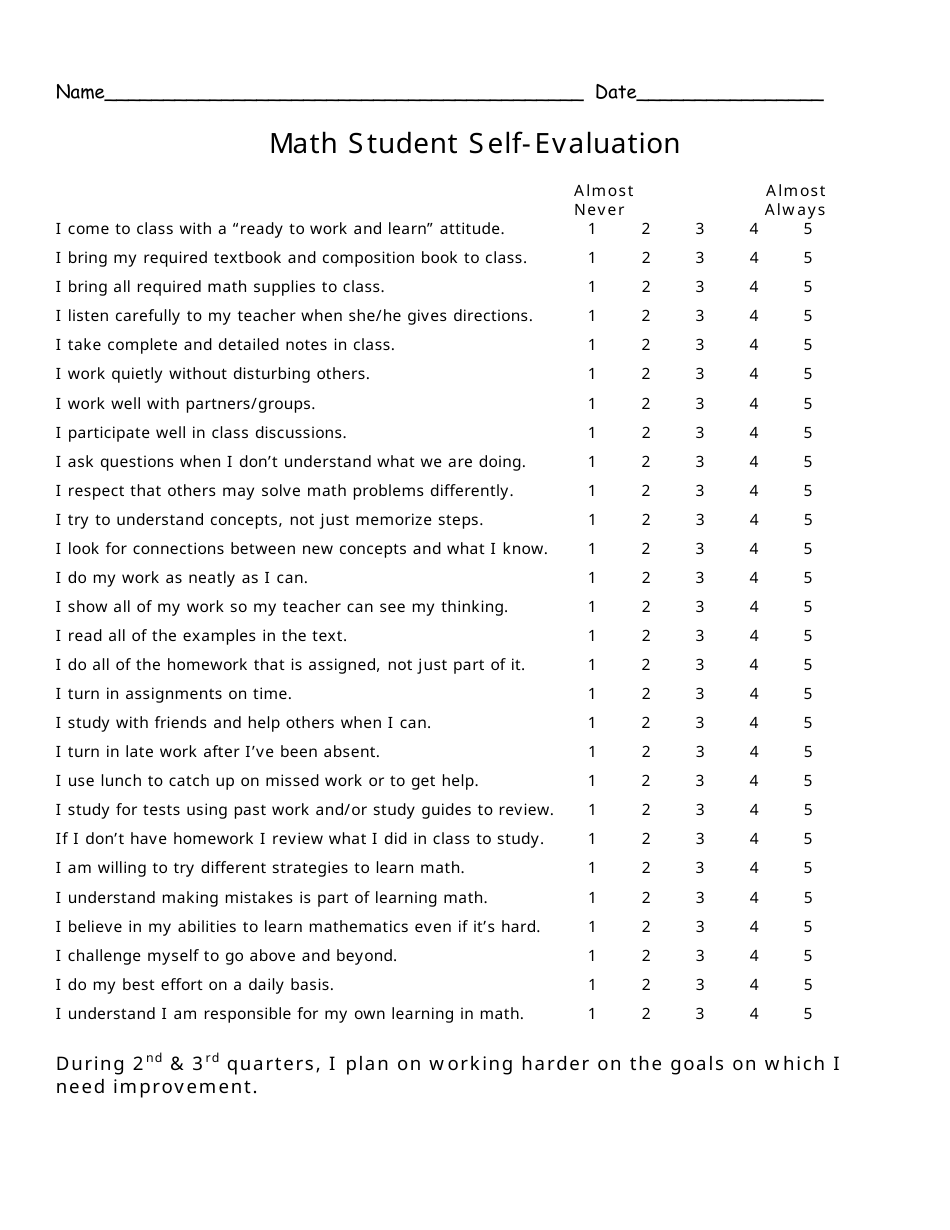# Math Student Self-evaluation Form

The Math Student Self-evaluation Form is a tool to assess a student's understanding and progress in mathematics. It allows students to reflect on their strengths and weaknesses, set goals, and communicate their needs to teachers.

The Math Student Self-evaluation Form is typically filed by the math student themselves.

## FAQ

Q: What is a math student self-evaluation form?
A: A math student self-evaluation form is a tool that allows students to assess their own math skills and progress.

Q: Why would a math student use a self-evaluation form?
A: A math student may use a self-evaluation form to reflect on their learning, identify areas for improvement, and set goals for their mathematical development.

Q: What can a math student learn from a self-evaluation form?
A: A math student can learn about their strengths and weaknesses in math, as well as any misconceptions or gaps in their understanding.

Q: Can a math student use a self-evaluation form to track their progress?
A: Yes, a math student can use a self-evaluation form to track their progress over time and monitor their improvement in various math skills.

Q: Are math self-evaluation forms helpful for teachers?
A: Yes, math self-evaluation forms can provide valuable insights for teachers about their students' perceptions of their own math abilities and learning needs.

•